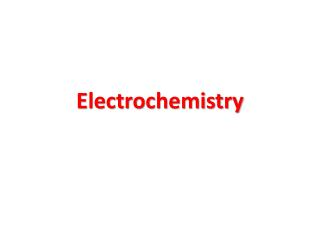Download PresentationElectrochemistry

# Electrochemistry - PowerPoint PPT PresentationDownload Presentation## Electrochemistry

- - - - - - - - - - - - - - - - - - - - - - - - - - - E N D - - - - - - - - - - - - - - - - - - - - - - - - - - -
##### Presentation Transcript

1. Electrochemistry

2. Electrolysis and Electrical Conductance - Electrolytes and Non-electrolytes - Electrolysis and it’s mechanism • Electrical Units • Coulomb • Ampere • Ohm • Volt

3. Faraday’s Law of Electrolysis First Law: The amount of a given product liberated at an electrode during electrolysis is directly proportional to the quantity of electricity which passes through the electrolyte solution. Second Law: When the same quantity of electricity passes through solutions of different electrolytes, the amounts of the substances liberated at the electrodes are directly proportional to their chemical equivalents.

4. The electrical Unit Faraday The quantity of electricity required to liberate one gram-equivalent of a substance is 96,500 coulombs. This quantity of electricity is known as Faraday (F). 1 Faraday = 96, 500 coulombs = 1 Mole electrons

5. Conductance or Conductivity The power of electrolytes to conduct electric currents is termed as conductivity or conductance. I = E / R, where I= current flow, E=potential differences at two ends, R=resistance Specific resistance: R α l / A R = ρ x l/A

6. Specific conductance: The conductance of one centimeter cube (cc) of a solution of an electrolyte. Κ = 1 / ρ Equivalent conductance The conductance of an electrolyte obtained by dissolving one gram-equivalent of it V cc of water. Λ = κ x V

7. Molar conductance The conduction of all ions produced by one mole (one gram-molecular weight) of an electrolyte when dissolved in a certain volume V cc. μ = κ x V

8. Molar Conductance ( Unit)

9. Kohlrausch’s Law The equivalent conductance of an electrolyte at infinite dilution is equal to the sum of the equivalent conductances of the component ions. λ∞ = λa + λc

10. Ostwald’s Dilution Law Limitation: Holds good only for weak electrolytes and fails completely when applied to strong electrolytes.

11. Electrochemical Reactions In electrochemical reactions, electrons are transferred from one species to another. Electrochemical Cells A device for producing an electrical current from a chemical reaction (redox Reaction) is called an electrochemical cell. How a Redox reaction can produce an electrical current?

12. Electromotive Force (emf) • Water only spontaneously flows one way in a waterfall. • Likewise, electrons only spontaneously flow one way in a redox reaction—from higher to lower potential energy.

13. = Ered (cathode) −Ered (anode)  Ecell  • The potential difference between the anode and cathode in a cell is called the electromotive force (emf). • It is also called the cell potential, and is designated Ecell.

14. Single electrode potential: The potential of a single electrode in a half-cell is called the single electrode potential. Standard EMF of a cell (Eᴼ) The emf of a cell with 1 M solutions of reactants and products In solution measured at 25 ᴼC.

15. Voltaic Cells A voltaic cell is one in which electrical current is generated By a spontaneous redox reaction. • Predicting the oxidizing or reducing ability • Predicting whether a metal will displace another metal • from it salt or not

16. THE NERNST EQUATION

17. Multiple Choice Questions Question 1. The conductivity of an electrolyte is due to the Presence of ions in the electrolyte free movement of ions in the solution reunion of ions in the solution release of heat energy due to ionisation Key : (b)

18. Question2. The electrical conductivity of an electrolyte depends upon The number of molecules in the electrolyte the number of ions present in the electrolyte the number ions present in the solution the number of molecules of the solvent Key: (c)

19. Question 3. Ostwald’s dilution law is applicable to All electrolytes strong electrolytes weak electrolytes non-electrolytes Key (C)

20. Question 4. The site of oxidation in an electrochemical cell is The anode the cathode the electrode the salt bridge Key : (a)

21. Question 5. The salt bridge in the electrochemical cell serves to Increase the rate at which equilibrium is attained increase the voltage of the cell maintain electrical neutrality increase the oxidation/reduction rate Key : (C)